# SAT Math Multiple Choice Question 824: Answer and Explanation

### Test Information

Question: 824

14. The earth is made up of four primary layers: the inner core, outer core, mantle, andcrust. The outer core is more than 800 miles and less than 2,200 miles from the Earth'scenter. Which of the following inequalities represents all possible distances, d, in miles, from the Earth's center that are in the outer core?

• A.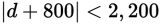• B.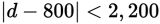• C.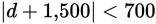• D.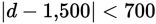Getting to the Answer: The question states that the outer core is more than 800 miles and less than 2,200 miles from the Earth's center, so the possible distances from the Earth's center that are in the outer core are given by the interval 800 < d < 2,200. the midpoint of this interval is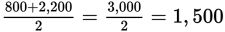. The interval 800 < d < 2,200 consists of all the points that are within 700 units (1,500 - 800 = 700 and 2,200 - 1,500 = 700) of the midpoint. Thus, the equivalent absolute value inequality is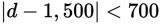, which is (d).